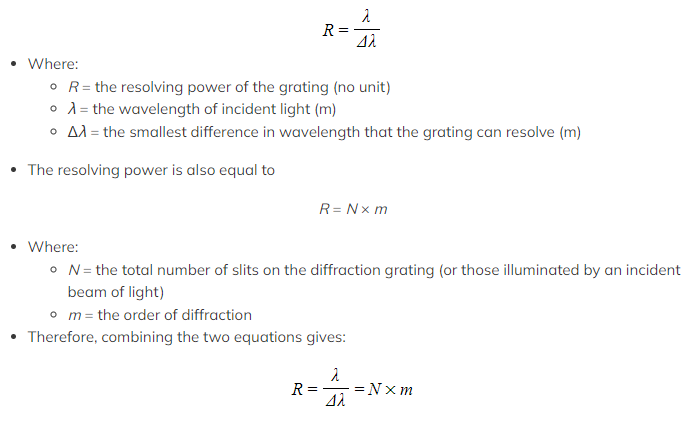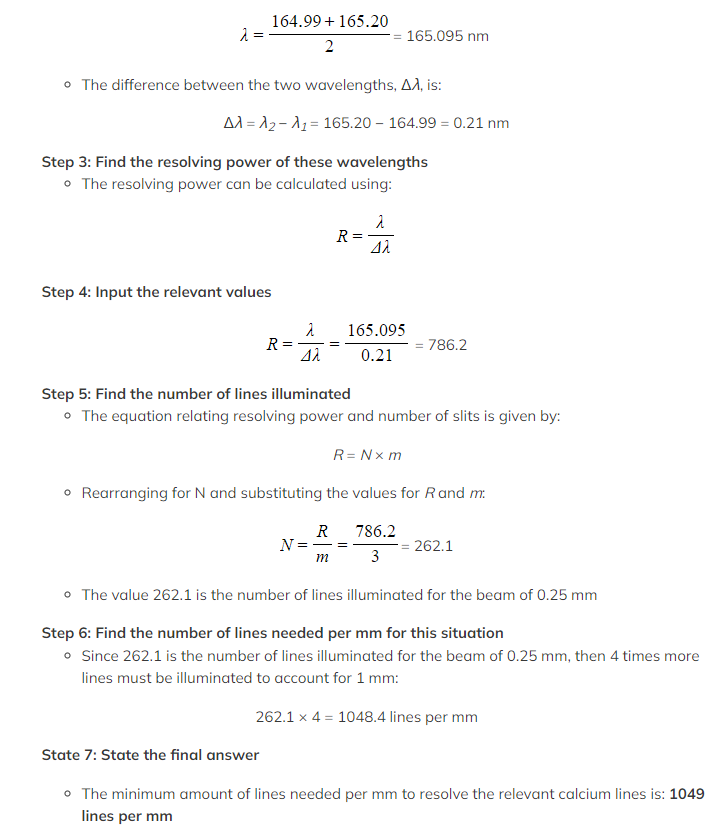# IB DP Physics: HL复习笔记9.4.3 Resolvance of Diffraction Gratings

### Resolvance of Diffraction Gratings

• In order to know if a diffraction grating is able to resolve two wavelengths, the resolving power of the diffraction grating must be found
• This is based on the Rayleigh criterion as applied to diffraction gratings and their output
• The resolving power, R, of a diffraction grating is given by:Worked Example

A student is using a diffraction grating to resolve two emission wavelengths from calcium in the 3rd order of the spectrum. These wavelengths are 164.99 nm and 165.20 nm.

Determine the minimum number of lines per mm needed if a beam of width 0.25 mm is incident upon the diffraction grating.

Step 1: List the known values
• Order of diffraction, m = 3
• Wavelength, λ1 = 164.99 nm
• Wavelength, λ2 = 165.20 nm
• Beam width = 0.25 mm
Step 2: Determine values for λ and Δλ
• The value of the incident wavelength, λ, can be determined from the mean of the two wavelengths:#### Exam Tip

In the worked example, the answer may look as though it has been rounded incorrectly but we are looking for the actual number of lines here, not fractions of lines

Rounding down to 1048 would leave the grating nearly half a line short, which can't happen, so always round up to the nearest whole number